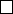8Matrix groups

IA Groups8.2 Actions of GL
n
(C)
Proposition. GL
n
(
C
) acts faithfully on
C
n
by left multiplication to the vector,
with two orbits (0 and everything else).
Proof. First show that it is a group action:
1. If A GL
n
(C) and v C
n
, then Av C
n
. So it is closed.
2. Iv = v for all v C
n
.
3. A(Bv) = (AB)v.
Now prove that it is faithful: a linear map is determined by what it does
on a basis. Take the standard basis
e
1
= (1
,
0
, · · · ,
0)
, · · · e
n
= (0
, · · · ,
1). Any
matrix which maps each
e
k
to itself must be
I
(since the columns of a matrix
are the images of the basis vectors)
To show that there are 2 orbits, we know that
A0
=
0
for all
A
. Also, as
A
is invertible,
Av
=
0 v
=
0
. So
0
forms a singleton orbit. Then given any
two vectors
v 6
=
w C
n
\ {
0
}
, there is a matrix
A GL
n
(
C
) such that
Av
=
w
(cf. Vectors and Matrices).
Similarly, GL
n
(R) acts on R
n
.
Proposition. GL
n
(C) acts on M
n×n
(C) by conjugation. (Proof is trivial)
This action can be thought of as a “change of basis” action. Two matrices
are conjugate if they represent the same map but with respect to different bases.
The P is the base change matrix.
From Vectors and Matrices, we know that there are three different types of
orbits for GL
2
(C): A is conjugate to a matrix of one of these forms:
(i)
λ 0
0 µ
, with λ 6= µ, i.e. two distinct eigenvalues
(ii)
λ 0
0 λ
, i.e. a repeated eigenvalue with 2-dimensional eigenspace
(iii)
λ 1
0 λ
, i.e. a repeated eigenvalue with a 1-dimensional eigenspace
Note that we said there are three types of orbits, not three orbits. There are
infinitely many orbits, e.g. one for each of λI.